Stokes Law Calculators Enter value, select unit and click on calculate. Result will be displayed.

Calculate Terminal Velocity (Fall Velocity or Settling Velocity)
Calculate Acceleration Of Gravity.
Calculate Diameter Of a Particle
Calculate Density Of Medium
Calculate Density Of Particle
Calculate Viscosity Of Medium

 Web www.calculatoredge.com

Calculate Terminal Velocity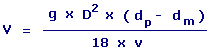V = Terminal Velocity D = Diameter Of a Particle g = Acceleration Of Gravity v = Viscosity Of Medium dp = Density Of Particle dm = Density Of Medium

 Enter your values: Acceleration Of Gravity: Meter / Second^2 Centimeter / Second^2 Foot / Second^2 Inch / Second^2 Kilometer / Second^2 Mile / Second^2 Millimeter / Second^2 Yard / Second^2 Diameter Of a Particle: Meter Centimeter Dekameter Decimeter Exameter Femtometer Foot Hectometer Inch Kilometer Megameter Micrometer Mile Millimeter Nanometer Petameter Picometer Yard Density Of Particle: Gram / Meter^3 Gram / Centimeter^3 Pound / Foot^3 Kilogram / Meter^3 Milligram / Liter Density Of Medium: Gram / Meter^3 Gram / Centimeter^3 Pound / Foot^3 Kilogram / Meter^3 Milligram / Liter Viscosity Of Medium: Gram / Meter-Second Kilogram / Meter-Second Kilogram / Meter-Hour Result: Terminal Velocity: Meter/Second

Calculate Acceleration Of Gravity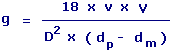g = Acceleration Of Gravity V = Terminal Velocity v = Viscosity Of MediumD = Diameter Of a Particle dp = Density Of Particle dm = Density Of Medium

 Enter your values: Terminal Velocity : Meter / Second Foot / Second Centimeter / Hour Centimeter / Second Foot / Day Inch / Hour Inch / Second Kilometer / Second Knot Meter / Day Mile / Hour Mile / Second Millimeter / Second Yard / Second Diameter Of a Particle: Meter Centimeter Attometer Dekameter Decimeter Exameter Femtometer Foot Hectometer Inch Kilometer Megameter Micrometer Mile Millimeter Nanometer Petameter Picometer Yard Density Of Particle: Gram / Meter^3 Gram / Centimeter^3 Pound / Foot^3 Kilogram / Meter^3 Milligram / Liter Density Of Medium: Gram / Meter^3 Gram / Centimeter^3 Pound / Foot^3 Kilogram / Meter^3 Milligram / Liter Viscosity Of Medium: Gram / Meter-Second Kilogram / Meter-Second Kilogram / Meter-Hour Result: Acceleration Of Gravity: Meter/Second2

Calculate Diameter Of a Particle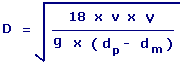D = Diameter Of a Particle V = Terminal Velocity v = Viscosity Of Medium g = Acceleration Of Gravity dp = Density Of Particle dm = Density Of Medium

 Enter your values: Viscosity Of Medium: Gram / Meter-Second Kilogram / Meter-Second Kilogram / Meter-Hour Terminal Velocity : Meter / Second Foot / Second Centimeter / Hour Centimeter / Second Foot / Day Inch / Hour Inch / Second Kilometer / Second Knot Meter / Day Mile / Hour Mile / Second Millimeter / Second Yard / Second Acceleration Of Gravity: Meter / Second^2 Centimeter / Second^2 Foot / Second^2 Inch / Second^2 Kilometer / Second^2 Mile / Second^2 Millimeter / Second^2 Yard / Second^2 Density Of Particle: Gram / Meter^3 Gram / Centimeter^3 Pound / Foot^3 Kilogram / Meter^3 Milligram / Liter Density Of Medium: Gram / Meter^3 Gram / Centimeter^3 Pound / Foot^3 Kilogram / Meter^3 Milligram / Liter Result: Diameter Of a Particle: Meter

Calculate Density Of Medium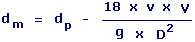dm = Density Of Medium dp = Density Of Particle V = Terminal Velocity v = Viscosity Of Medium D = Diameter Of a Particle g = Acceleration Of Gravity

 Enter your values: Density Of Particle: Gram / Meter^3 Gram / Centimeter^3 Pound / Foot^3 Kilogram / Meter^3 Milligram / Liter Viscosity Of Medium: Gram / Meter-Second Kilogram / Meter-Second Kilogram / Meter-Hour Terminal Velocity : Meter / Second Foot / Second Centimeter / Hour Centimeter / Second Foot / Day Inch / Hour Inch / Second Kilometer / Second Knot Meter / Day Mile / Hour Mile / Second Millimeter / Second Yard / Second Acceleration Of Gravity: Meter / Second^2 Centimeter / Second^2 Foot / Second^2 Inch / Second^2 Kilometer / Second^2 Mile / Second^2 Millimeter / Second^2 Yard / Second^2 Diameter Of a Particle: Meter Centimeter Attometer Dekameter Decimeter Exameter Femtometer Foot Hectometer Inch Kilometer Megameter Micrometer Mile Millimeter Nanometer Petameter Picometer Yard Result: Density Of Medium: Gram/Meter3

Calculate Density Of Particle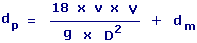dp = Density Of Particle dm = Density Of MediumV = Terminal Velocity v = Viscosity Of Medium D = Diameter Of a Particle g = Acceleration Of Gravity

 Enter your values: Density Of Medium: Gram / Meter^3 Gram / Centimeter^3 Pound / Foot^3 Kilogram / Meter^3 Milligram / Liter Viscosity Of Medium: Gram / Meter-Second Kilogram / Meter-Second Kilogram / Meter-Hour Terminal Velocity : Meter / Second Foot / Second Centimeter / Hour Centimeter / Second Foot / Day Inch / Hour Inch / Second Kilometer / Second Knot Meter / Day Mile / Hour Mile / Second Millimeter / Second Yard / Second Acceleration Of Gravity: Meter / Second^2 Centimeter / Second^2 Foot / Second^2 Inch / Second^2 Kilometer / Second^2 Mile / Second^2 Millimeter / Second^2 Yard / Second^2 Diameter Of a Particle: Meter Centimeter Attometer Dekameter Decimeter Exameter Femtometer Foot Hectometer Inch Kilometer Megameter Micrometer Mile Millimeter Nanometer Petameter Picometer Yard Result: Density Of Particle: Gram/Meter3

Calculate Viscosity Of Medium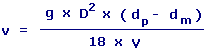v = Viscosity Of Medium dp = Density Of Particle dm = Density Of Medium D = Diameter Of a Particle g = Acceleration Of Gravity V = Terminal Velocity

 Enter your values: Acceleration Of Gravity: Meter / Second^2 Centimeter / Second^2 Foot / Second^2 Inch / Second^2 Kilometer / Second^2 Mile / Second^2 Millimeter / Second^2 Yard / Second^2 Diameter Of a Particle: Meter Centimeter Attometer Dekameter Decimeter Exameter Femtometer Foot Hectometer Inch Kilometer Megameter Micrometer Mile Millimeter Nanometer Petameter Picometer Yard Density Of Particle: Gram / Meter^3 Gram / Centimeter^3 Pound / Foot^3 Kilogram / Meter^3 Milligram / Liter Density Of Medium: Gram / Meter^3 Gram / Centimeter^3 Pound / Foot^3 Kilogram / Meter^3 Milligram / Liter Terminal Velocity : Meter / Second Foot / Second Centimeter / Hour Centimeter / Second Foot / Day Inch / Hour Inch / Second Kilometer / Second Knot Meter / Day Mile / Hour Mile / Second Millimeter / Second Yard / Second Result: Viscosity Of Medium: Gram/Meter-SecondLINKSDISCLAIMERCONTACT US